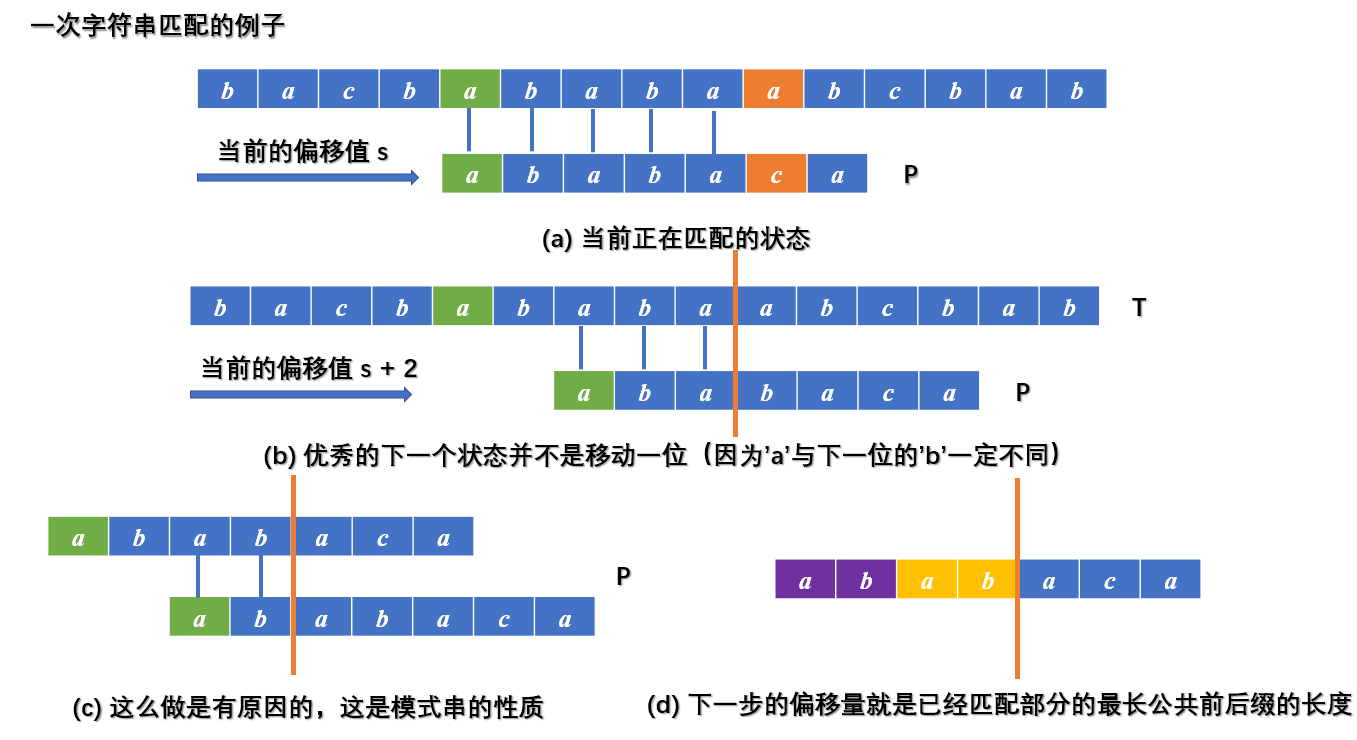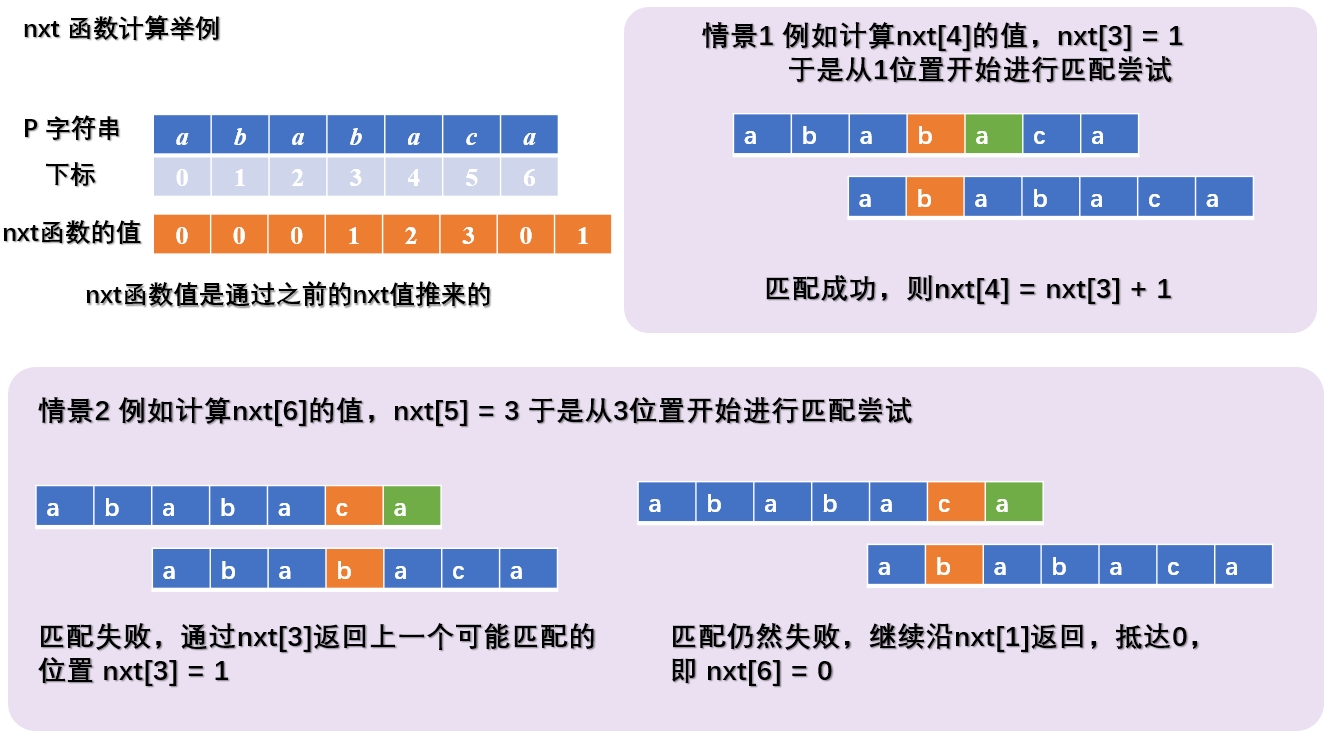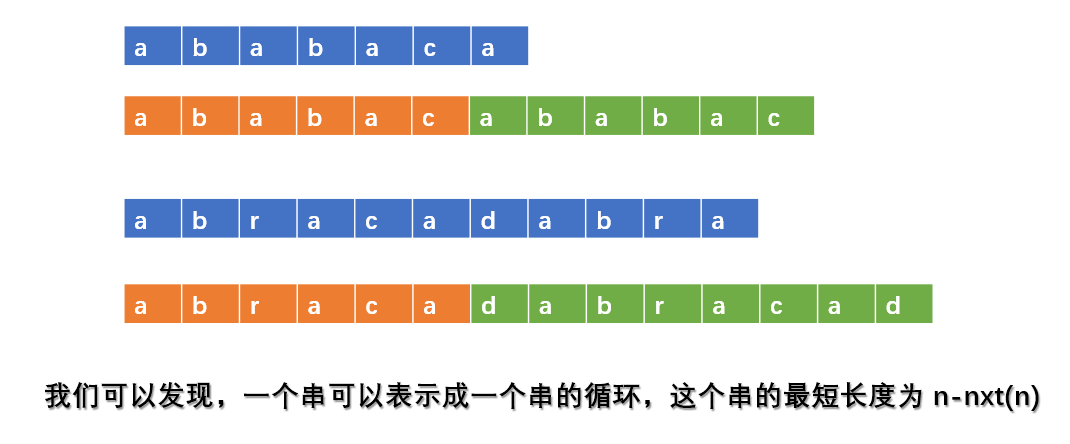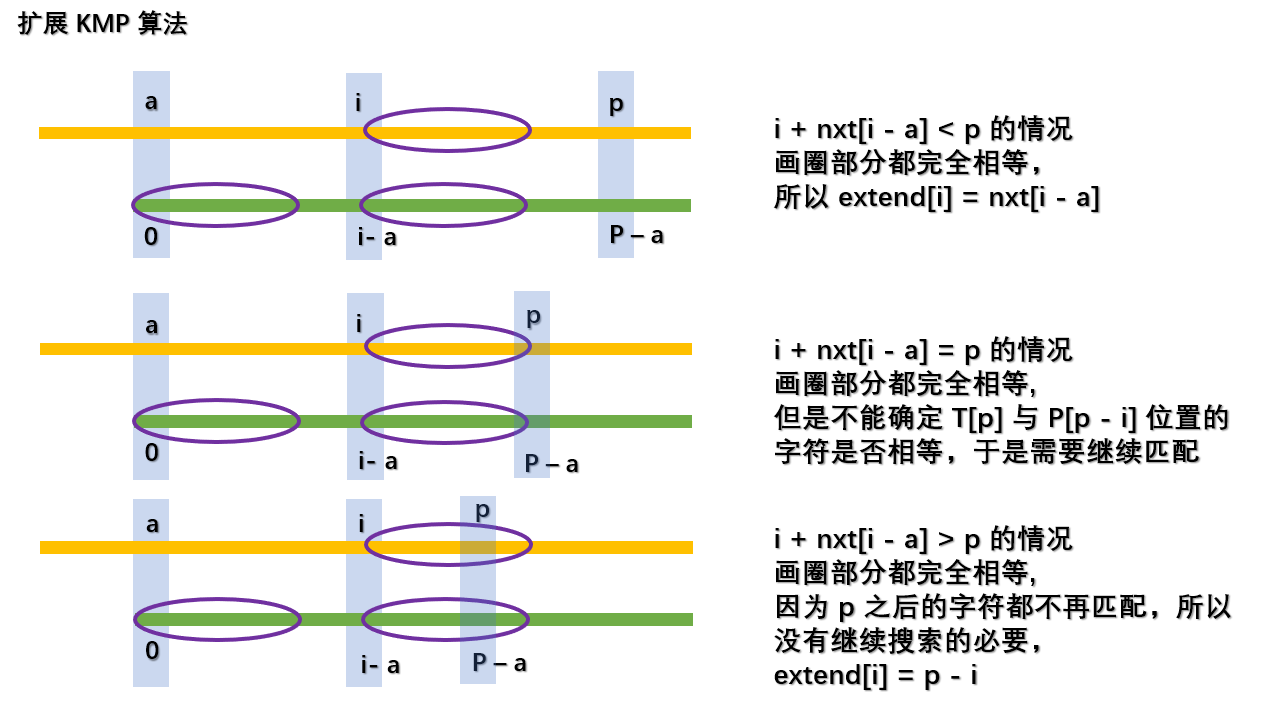# KMP 模式匹配算法与扩展 KMP 相关

## 前言

### 本文导览

KMP 算法简称 Knuth-Morris-Pratt Algorithm (中译：克努特——莫里斯——普拉特操作)， 主要用于解决字符串中的模式匹配问题，本文将介绍以下几点

• 字符串的模式匹配问题
• nxt 辅助数组的构建
• KMP 算法的实现

exKMP 算法是对 KMP 算法的一个拓展，使得在同样的时间复杂度内，可以获得更多的信息，本文将介绍以下几点

• exKMP 所要解决的问题
• exKMP 算法的实现

【字符集大小】

【字符串的连接】

【前缀与后缀】

## KMP 模式匹配算法

### 模式匹配问题### 充分挖掘模式串的性质### nxt 辅助数组的构建

inline void getFail(char *P, int *nxt)
{
int m = strlen(P);
nxt = 0; nxt = 0;
for(int i = 1; i < m; i++)
{
int j = nxt[i];
while(j != 0 && P[i] != P[j]) j = nxt[j];
nxt[i + 1] = P[i] == P[j] ? j + 1 : 0;
}
}### 通过 nxt 辅助数组来进行模式匹配

inline void find(char *T, char *P, int *nxt)
{
int n = strlen(T), m = strlen(P);
getFail(P, nxt);
int j = 0;
for(int i = 0; i < n; i++)
{
while(j != 0 && P[j] != T[i]) j = nxt[j];
if(P[j] == T[i]) j++;
if(j == m) \\ready_find
}
}

### nxt 数组的其他性质

• 根据 nxt 数组的性质，长度为 $n$ 的字符串的最长公共前后缀（不包括自己）的长度为 $nxt(n)$
• 根据上一条性质，我们可以发现，一个串可以表示成一个子串的循环，这个子串的最短长度为 $n-nxt(n)$，下面这张图帮助你的理解。## exKMP 扩展 KMP 算法

exKMP 算法是对 KMP 算法的扩展，它不仅可以实现 KMP 的功能，还可以得到更优秀的字符串信息。在开始下面的阅读之前，需要读者已经掌握上面介绍的 KMP 算法。

### 问题的提出

exKMP 算法要求我们求解出一个关于字符串 $T$$P$ 的一个数组 extendextend 表示对于 $T$ 的每一个后缀，与 $P$ 串的最长公共前缀的长度，即，从第 i 为开始所能匹配到的最远位置。

### 尝试解决

• 位置标记 $a$
• 位置标记 $p$
• 辅助数组 $nxt$inline void getFail(char *P, int *nxt)
{
int a = 0, p = 0, m = strlen(P);
nxt = m;
for(int i = 1; i < m; i++)
{
if(i + nxt[i - a] >= p)
{
p = max(p, i);
while(p < m && P[p] == P[p - i]) p++;
nxt[i] = p - i; a = i;
}
else nxt[i] = nxt[i - a];
}
}
inline void getExtend(char *T, char *P, int *nxt, int *extend)
{
int a = 0, p = 0;
int m = strlen(P), n = strlen(T);
getFail(T, nxt);
for(int i = 0; i < n; i++)
{
if(i + nxt[i - a] >= p)
{
p = max(i, p);
while(p < n && p - i < m && T[p] == P[p - i]) p++;
extend[i] = p - i; a = i;
}
else extend[i] = nxt[i - a];
}
}

## 算法的时间复杂度

### KMP 算法的时间复杂度

KMP 算法由初始化和进行匹配两部分进行，对于初始化算法部分，我们来证明每一次的 while 总共会进行 $O(|P|)$ 次，首先：每一次 nxt[i] 的增长最多是 1，也就是最多增长 $|P|$ 次，其次：每一次 while 循环只会降低 nxt[i] 的值而不会增加。综合这些因素，递减来源于 while 循环，最多下降 $|P|$ 次，所以复杂度为 $O(|P|)$。这只是一个大致的感性理解，有兴趣的读者可以使用部分摊还分析的知识。并且类比于初始化操作，匹配的复杂度是 $O(|T|)$

KMP 算法预处理 $O(|P|)$，匹配 $O(|T|)$，是优秀的线性时间算法。

### exKMP 算法的时间复杂度分析

KMP 算法预处理 $O(|P|)$，匹配 $O(|T|)$，也是优秀的线性时间算法。

## 后记

exKMP算法的优秀讲解

KMP与MP相关